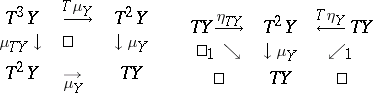# Standard construction

A concept in category theory. Other names are triple, monad and functor-algebra.

Let $\mathfrak{S}$ be a category. A standard construction is a functor $T : \mathfrak{S} \to \mathfrak{S}$ equipped with natural transformations $\eta : \operatorname{Id} \to T$ and $\mu : T^2 \to T$ such that the following diagrams commute:The basic use of standard constructions in topology is in the construction of various classifying spaces and their algebraic analogues, the so-called bar-constructions.